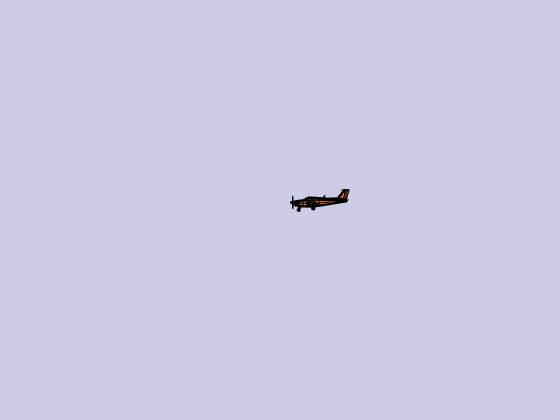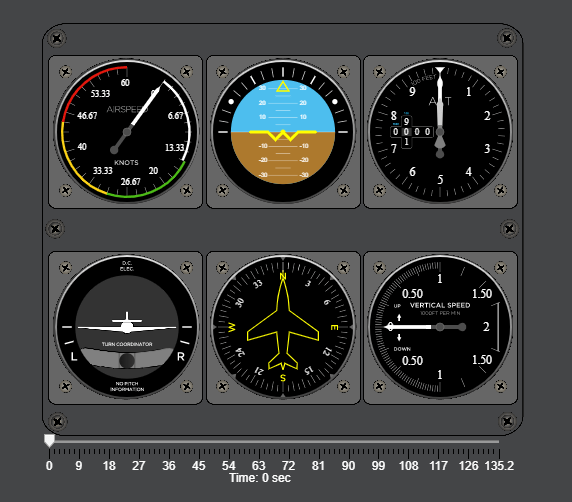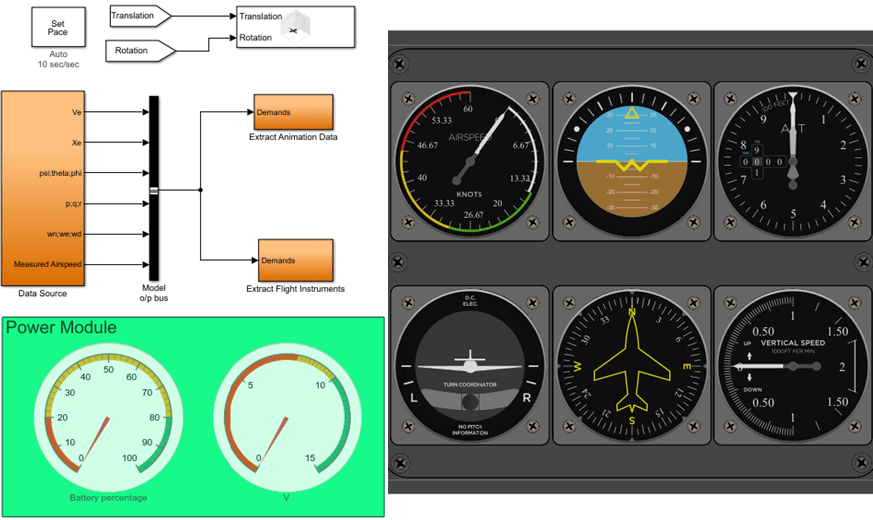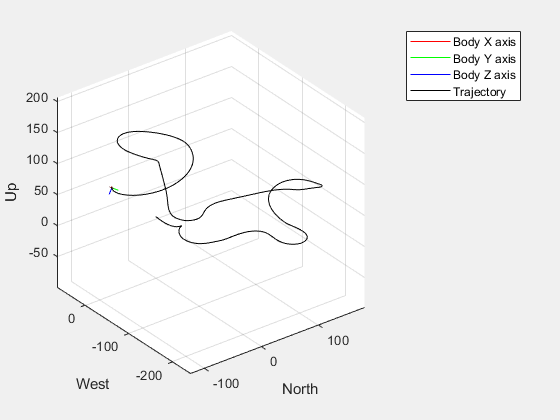# Flight Instrument Gauge Visualization for a Drone

Import and visualize a drone flight log using 3-D animations and flight intrument gauges. This example obtains a high level overview of flight performance in MATLAB® using Flight Instruments (Aerospace Toolbox) functions in Aerospace Toolbox™. Then, to view signals in a custom interface in Simulink® , the example uses the Flight Instruments (Aerospace Blockset) blocks from Aerospace Blockset™

The example extracts the signals of interest from a ULOG file and plays back the UAV flight trajectory in MATLAB. Then, those signals are replayed in a Simulink model using instrument blocks.

### Import a Flight log

A drone log file records information about the flight at regular time intervals. This information gives insight into the flight performance. Flight instrument gauges display navigation variables such as attitude, altitude, and heading of the drone. The ULOG log file for this example was obtained from an airplane model running in the Gazebo simulator.

Import the logfile using `ulogreader`. Create a `flightLogSignalMapping` object for ULOG files.

To understand the convention of the signals, the units, and their reference frame, inspect the information within the `plotter` object. This information about units within log file becomes important when connecting the signals to flight instrument gauges.

```data = ulogreader("fwflight.ulg"); plotter = flightLogSignalMapping("ulog"); info(plotter,"Signal")```
```ans=14×4 table SignalName IsMapped SignalFields FieldUnits _____________________ ________ __________________________________________________________________________________________________________________________________________________________________________________________________________ ___________________________________________________ "Accel" true "AccelX, AccelY, AccelZ" "m/s^2, m/s^2, m/s^2" "Airspeed" true "PressDiff, IndicatedAirSpeed, Temperature" "Pa, m/s, degreeC" "AttitudeEuler" true "Roll, Pitch, Yaw" "rad, rad, rad" "AttitudeRate" true "BodyRotationRateX, BodyRotationRateY, BodyRotationRateZ" "rad/s, rad/s, rad/s" "AttitudeTargetEuler" true "RollTarget, PitchTarget, YawTarget" "rad, rad, rad" "Barometer" true "PressAbs, PressAltitude, Temperature" "Pa, m, degreeC" "Battery" true "Voltage_1, Voltage_2, Voltage_3, Voltage_4, Voltage_5, Voltage_6, Voltage_7, Voltage_8, Voltage_9, Voltage_10, Voltage_11, Voltage_12, Voltage_13, Voltage_14, Voltage_15, Voltage_16, RemainingCapacity" "v, v, v, v, v, v, v, v, v, v, v, v, v, v, v, v, %" "GPS" true "Latitude, Longitude, Altitude, GroundSpeed, CourseAngle, SatellitesVisible" "degree, degree, m, m/s, degree, N/A" "Gyro" true "GyroX, GyroY, GyroZ" "rad/s, rad/s, rad/s" "LocalNED" true "X, Y, Z" "m, m, m" "LocalNEDTarget" true "XTarget, YTarget, ZTarget" "m, m, m" "LocalNEDVel" true "VX, VY, VZ" "m/s, m/s, m/s" "LocalNEDVelTarget" true "VXTarget, VYTarget, VZTarget" "m/s, m/s, m/s" "Mag" true "MagX, MagY, MagZ" "Gs, Gs, Gs" ```

### Extract Signals of Interest

To visualize the drone flight using instrument gauges, extract the attitude, position, velocity, and airspeed at each timestep. Specify the appropriate signal name from the info table in the previous step. Call the `extract` function with the appropriate signal names. The time vector element of signals are adjusted so they start at 0 seconds.

```% Extract attitude and roll-pitch-yaw data. rpy = extract(plotter, data,"AttitudeEuler"); rpy{1}.Time=rpy{1}.Time-rpy{1}.Time(1); RollData = timetable(rpy{1}.Time,rpy{1}.Roll,... 'VariableNames',{'Roll'}); PitchData = timetable(rpy{1}.Time,rpy{1}.Pitch,... 'VariableNames',{'Pitch'}); YawData = timetable(rpy{1}.Time,rpy{1}.Yaw,... 'VariableNames',{'Yaw'}); % Extract position and xyz data. Position = extract(plotter, data,"LocalNED"); Position{1}.Time = Position{1}.Time-Position{1}.Time(1); X = timetable(Position{1}.Time,Position{1}.X,... 'VariableNames',{'X'}); Y = timetable(Position{1}.Time,Position{1}.Y,... 'VariableNames',{'Y'}); Z = timetable(Position{1}.Time,Position{1}.Z,... 'VariableNames',{'Z'}); % Extract velocity data. vel = extract(plotter, data,"LocalNEDVel"); vel{1}.Time=vel{1}.Time-vel{1}.Time(1); XVel = timetable(vel{1}.Time,vel{1}.VX,... 'VariableNames',{'VX'}); YVel = timetable(vel{1}.Time,vel{1}.VY,... 'VariableNames',{'VY'}); ZVel = timetable(vel{1}.Time,vel{1}.VZ,... 'VariableNames',{'VZ'}); % Extract Airspeed magnitude data. airspeed = extract(plotter, data,"Airspeed"); Airspeed = timetable(airspeed{1}.Time,airspeed{1}.IndicatedAirSpeed,... 'VariableNames',{'Airspeed'}); ```

### Convert Units and Preprocess Data for Gauges

Our flight log records data in SI Units. The flight instrument gauges require a conversion to Aerospace Standard Unit System represented by English System. This conversion is handled in the visualization block available in attached Simulink model for the user. The turn coordinator indicates the yaw rate of the aircraft using an indicative banking motion (which differs from the bank angle). In order to compute the yaw rate, convert the angular rates from body frame to vehicle frame as given below:

`$\stackrel{˙}{\psi }=\frac{\mathrm{qcos}\left(\varphi \right)+\mathrm{rsin}\left(\varphi \right)}{\mathrm{cos}\theta }$`

The inclinometer ball within turn coordinator indicates the sideslip of the aircraft. This sideslip angle is based on the angle between the body of the aircraft and computed airspeed. For an accurate airspeed, a good estimate of velocity and wind vector is required. Most small UAV's do not possess sensors to estimate wind vector data or airspeed while flying. UAV's can face between 20-50% of their airspeed in the form of crosswinds.

${\mathit{V}}_{\mathit{g}}$- ${\mathit{V}}_{\mathit{w}}$=${\mathit{V}}_{\mathit{a}}$

To compute sideslip and turn, extract wind and attitude rate data directly from the log file.

```% Extract roll, pitch and yaw rates and an estimated windspeed. [p,q,r,wn,we] = helperExtractUnmappedData(data); % Merge timetables. FlightData = synchronize(X,Y,Z,RollData,PitchData,YawData,XVel,YVel,ZVel,p,q,r,Airspeed,wn,we,'union','linear'); % Assemble an array for the data. FlightDataArray = double([seconds(FlightData.Time) FlightData.X FlightData.Y FlightData.Z FlightData.Roll ... FlightData.Pitch FlightData.Yaw,FlightData.VX,FlightData.VY,... FlightData.VZ,FlightData.p,FlightData.q,FlightData.r,FlightData.Airspeed,FlightData.wn,FlightData.we]); % Ensure time rows are unique. [~,ind]=unique(FlightDataArray(:,1)); FlightDataArray=FlightDataArray(ind,:); % Preprocess time data to specific times. flightdata = double(FlightDataArray(FlightDataArray(:,1)>=0,1:end));```

### Visualize Standard Flight Instrument Data in MATLAB

To get a quick overview of the flight , use the animation interface introduced in the Display Flight Trajectory Data Using Flight Instruments and Flight Animation (Aerospace Toolbox) example. The helper function `helperDroneInstruments` creates an instrument animation interface.

`helperDroneInstruments;`The Airspeed indicator dial indicates the speed of the drone. The Artificial Horizon indicator reveals the attitude of the drone excluding yaw. The Altimeter and Climb Rate indicator reveal the altitude as recorded within the barometer and the climb rate sensors respectively. The Turn Coordinator indicates the yaw rate of the aircraft and sideslip. If the inclinometer skews towards left or right, this denotes a slip or skid situation. In a coordinated turn, the sideslip should be zero.

In Simulik, you can create custom visualizations of signals using intrument blocks to help diagnose problems with a flight. For example, voltage and battery data in log files can help diagnose failures due to inadequate power or voltage spikes. Extract this batter data below to visualize them.

```% Extract battery data. Battery = extract(plotter,data,"Battery"); % Extract voltage data from topic. Voltage = timetable(Battery{1}.Time,Battery{1}.Voltage_1,... 'VariableNames',{'Voltage_1'}); % Extract remaing battery capacity data from topic. Capacity = timetable(Battery{1}.Time,Battery{1}.RemainingCapacity,... 'VariableNames',{'RemainingCapacity'});```

Open the `'dronegauge'` model, which takes the loaded data and displays it on the different gauges and the UAV animation figure.

`open_system('dronegauges'); `Run the model. The generated figure shows the trajectory of the UAV in real time and the gauges show the current status of the flight.

`sim('dronegauges');`## SupportGet trial now